Tree Creation

 Stem_Levels number of branch levels level 0 = trunk; level 1 = main branches, growing out of the trunk; level 2 = sub branches growing out of the main branches; … last level = leaves growing out of the level before (stems are the trunk plus all branches)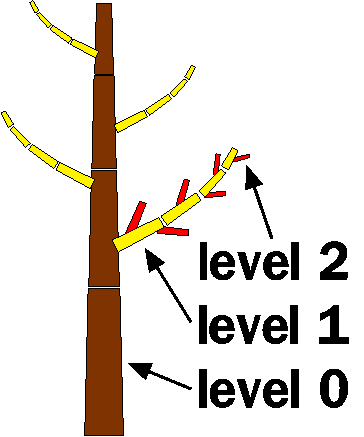N_Curve_Resolution number of segments the stems of level n are devided in 0_Curve_Resolution = x ® the trunk consists of (x+1) segments 1_Curve_Resolution = y ® each main branch consist of (y+1) segments ….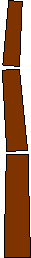0_Curve_Resolution = 3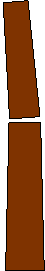0_CurveResolution = 2 N_Splits/Segment determines the number of splittings after each segment in level n if this value is decimal, the digits behind the point are added to the splits/segment value of the next segment.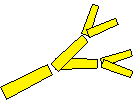1_Splits/Segment = 1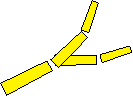1_Splits/Segment = 0.5 0_Base_Splits determines the number of splits right after the very first trunk segment all following splits are calculated with 0_splits_per_segment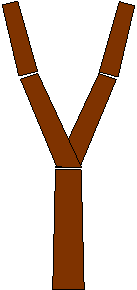0_Base_Splits = 1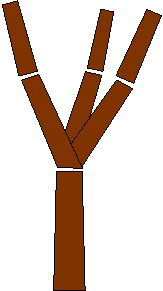0_Base_Splits = 2
 N_Split_Angle determines the angle between the longitudinal axis of the segments before and after a split the rotation around the longitudinal axis is partitioned evenly.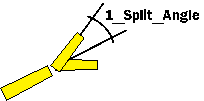Basesize height of the lowest main branch ® the part of the trunk beneath is branchless. 1_Branches stands for the count of main branches. their position on the trunk is evenly distributed between the end of basesize and the peak. (1_Branches = 4) 2_Branches determines a maximum for the count of sub branches. their position is equally distributed over each main branch. the number of branches is shortened by the length of the main branches. …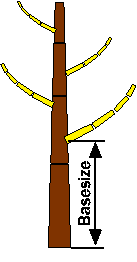N_Angle_Down determines the angle between the longitudinal axis of a stem and its sub stems this angle can be modified by the tree shape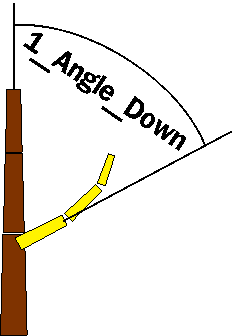N_Angle_Rotate determines the angle between two stems around the longitudinal axis of their parent stem.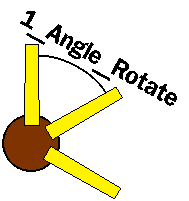(view from top) N_Curve determines the angle between the first and last segment of a stem in level N ® the angle between two segments is (n_curve / (n_curve_resolution + 1)).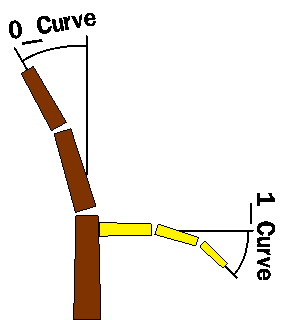N_Curve_Back if this value doesn’t equal zeor, n_curve is only distributed over the first half of segments and n_curve_back bends the second half.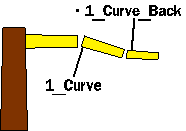0_Flare determines the widening of the base of the trunk. this effects the lower 1/8 of the trunk length.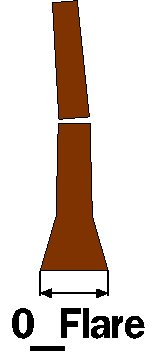0_Lobes determines the number of convexities. if this value is greater than zero, more triangles have to be drawn. otherwise the effect of lobes will no be visible. 0_Lobe_Depth determines the size of the convexities.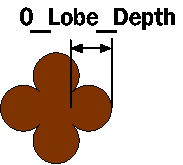(view from bottom) General_Scale height of the trunk in meters Radius_Ratio determines the radius of a stem in relation to its length (radius = length * radius_ratio) Ratio_Power determines the diameter of a branch in relation to its parent